## Gaussian Elimination

#### Introduction

We will now explore a more versatile way than the method of determinants to determine if a system of equations has a solution. We will indeed be able to use the results of this method to find the actual solution(s) of the system (if any). It should be noted that this method can be applied to systems of equations with an unequal number of equations and unknowns. However, we will only discuss systems with an equal number of equations and unknowns.

It is easiest to illustrate this method with an example. Consider the system of equations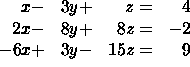To solve for x, y, and z we must eliminate some of the unknowns from some of the equations. Consider adding -2 times the first equation to the second equation and also adding 6 times the first equation to the third equation. The result is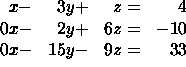We have now eliminated the x term from the last two equations. Now simplify the last two equations by dividing by 2 and 3, respectively: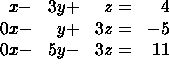To eliminate the y term in the last equation, multiply the second equation by -5 and add it to the third equation: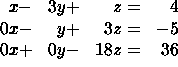The third equation says z=-2. Substituting this into the second equation yields y=-1. Using both of these results in the first equation gives x=3. The process of progressively solving for the unknowns is called back-substitution.

This is the essence of Gaussian elimination. However, we may clean up the notation in our work by using matrices.

#### Gaussian Elimination

Convert the system of equations above into an augmented matrix: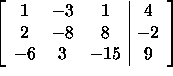This matrix contains all of the information in the system of equations without the x, y, and z labels to carry around. Now carry out the process outlined above. The notation to the right of each matrix describes the row operations that were performed to get the matrix on that line. For example 2R_1+R_2 -> R_2 means "replace row 2 with the sum of 2 times row 1 and row 2".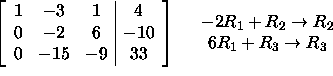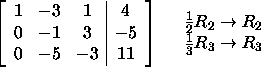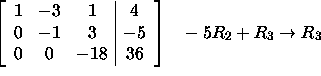If we now reverse the conversion process and turn the augmented matrix into a system of equations we haveWe can now easily solve for x, y, and z by back-substitution.

Remember: For a system of equations with a 3x3 matrix of coefficients, the goal of the process of Gaussian Elimination is to create (at least) a triangle of zeros in the lower left hand corner of the matrix below the diagonal. Note that you may switch the order of the rows at any time in trying to get to this form.

#### Example

Let's solve the following system of equations: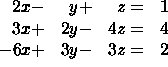In augmented matrix form we have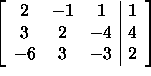We now use the method of Gaussian Elimination: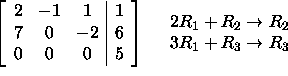We could proceed to try and replace the first element of row 2 with a zero, but we can actaully stop. To see why, convert back to a system of equations: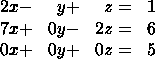Notice the last equation: 0=5. This is not possible. So the system has no solutions; it is not possible to find values x, y, and z that satisfy all three equations simultaneously.

#### Example

Solve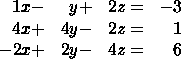In matrix form: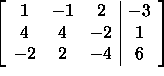Using Gaussian Elimination: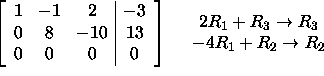Converting back to a system of equations: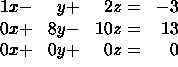Notice the last equation: 0=0 (this resulted from equation 3 being a linear combination of the other two equations). This is always true. And, we can solve the first two equations to get x and y as functions of z alone. Solving the second equation we get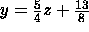And for the first equation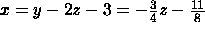The variable z in this problem is called a parameter since there are no constraints on what values z may take on. Thus, there are an infinite number of solutions - one for each value of z. Examples of solutions are (-11/8,13/8,0) and (-17/8,23/8,1) which come from setting z=0 and z=1, respectively. We may concisely write all solutions as triples of the form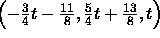where t is any real number.